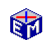Mean-Variance Analysis:  Risk vs. Expected Return

Mean-Variance Analysis quantifies the notions of risk and expected return by applying concepts from statistics.  The values of assets are taken to be random variables with various expected valuesModel Link:  Mean-Variance Analysis:  Risk vs. Expected Return
Printable PDF ExercisesThis application studies the case with one risky asset and one risk-free asset. The goal is to provide intuitive answers to three questions.

• How do agents arrive at their optimal portfolio?
• Why do different people will have different optimal portfolios?
• Why, even after they reach their optimal portfolio, do agents continue to trade?

The analysis is conducted within the framework of a diagram of risk vs. expected return.

Classic Economic Models

Macroeconomics

Introduction
Overview of Macro Models

Microeconomics

Introduction
Overview of Micro Models

Resources